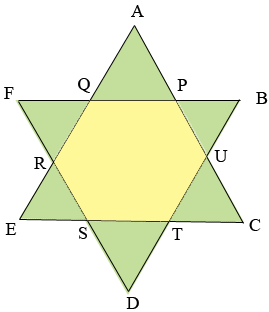SEARCH HOMEMath Central Quandaries & QueriesQuestion from Sunny: Triangles AEC and FDB are equilateral triangles and EA= DF= 12cm. The polygon at the center of the star is a regular hexagon. What is the area of the hexagon?Hi Sunny,

Is this the diagram?Since the hexagon is regular what do you know about the angles $UPQ$ and $PQR?$ What does that tell you about the triangle $AQP?$

To determine the area of the hexagon I suggest you use the method descried in Stephen's response to Scott.

PennyMath Central is supported by the University of Regina and The Pacific Institute for the Mathematical Sciences.